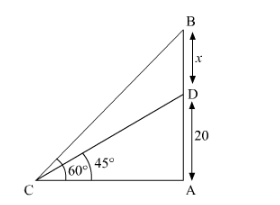# From a point on the ground,`
Question:

From a point on the ground, the angles of elevation of the bottom and top of a transmission tower fixed at the top of a 20 m high building are 45° and 60° respectively. Find height of the tower.

Solution:

It is given that tower is placed at a 20 m high building. The top and bottom of the tower makes an angle ofrespectively with the ground.

We have to find the height of the tower.Let DB is the tower

Height of the building = 20 m

Height of the tower = m

According to the figure,

$\frac{A B}{A C}=\tan 60^{\circ}$

$A C=\frac{A B}{\tan 60^{\circ}}$.......(1)

$\frac{A D}{A C}=\tan 45^{\circ}$

$A C=\frac{A D}{\tan 45^{\circ}} \ldots \ldots(2)$

Since (1) = (2)

$\frac{A B}{\tan 60^{\circ}}=\frac{A D}{\tan 45^{\circ}}$

$\frac{20+x}{\sqrt{3}}=\frac{20}{1}$

$20+x=20 \sqrt{3}$

$x=20 \sqrt{3}-20$

$x=20(\sqrt{3}-1)$

$x=20 \times 0.732$

$x=14.64 \mathrm{~m}$